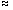Sums of Squares In
Unbalanced Analysis of Variance

 This page gives links to the following material by Donald Macnaughton about numerator sums of squares in unbalanced analysis of variance: Paper Which Sums of Squares Are Best In Unbalanced Analysis of Variance? Abstract Three fundamental concepts of science and statistics are entities, variables (which are formal representations of properties of entities), and relationships between variables. These concepts help to distinguish between two uses of the statistical tests in analysis of variance (ANOVA), namely to test for relationships between the response variable and the predictor variables in an experiment to test for relationships among the parameters of the model equation in an experiment. Two methods of computing ANOVA sums of squares are: Higher-level Terms are Omitted from the generating model equations (HTO = SPSS ANOVA EXPERIMENTALSAS Type IIBMDP4V with Weights are Sizes, wheresignifies "approximately equals") Higher-level Terms are Included in the generating model equations (HTI = SPSS ANOVA UNIQUE = SPSS MANOVA UNIQUE = SAS Type III = BMDP4V with Weights are Equal = BMDP2V = MINITAB GLM = SYSTAT MGLH = Data Desk Type 3). This paper evaluates the HTO and HTI methods of computing ANOVA sums for squares for fulfilling the two uses of the ANOVA statistical tests. Evaluation is in terms of the hypotheses being tested and relative power. It is concluded that (contrary to current practice) the HTO method is generally preferable when a researcher wishes to test the results of an experiment for evidence of relationships between variables. To Obtain This Paper This paper contains 22,000 words and 105 references. It is available in Adobe Portable Document Format (302 kilobytes) by clicking here. For information about the free PDF reader, click here. Click here if you have a problem viewing the PDF document. Computer Programs Computing Numerator Sums Of Squares In Unbalanced Analysis Of Variance The following documents and programs illustrate, in simple terms, the differences between five approaches to computing numerator sums of squares in unbalanced analysis of variance. The programs are written in SAS IML (Interactive Matrix Language) although a reader need not understand IML to understand the programs. The following documents are available: PR0139.HTM This document (115 kilobytes) contains output from SAS IML. It demonstrates the computation of three types sums of squares for all the standard effects in a 2 × 3 unbalanced experiment discussed by Shayle Searle (1987, 79). The material is heavily annotated. It is recommended that you read this document first. PR0165.HTM This document (35 kilobytes) contains output from SAS IML. It demonstrates the computation of five types of sums of squares for all the standard effects in a 4 × 3 × 2 unbalanced experiment discussed by Shayle Searle (1987, 392). The following SAS programs are available for downloading. (If a requested program file opens in your web browser, select "Save As" from the browser's File menu to save the file on a local drive.) PR0139.SAS This is the program that generated PR0139.HTM above. Download this file (52 kilobytes) if you wish to run the program on your computer. If you download this file, you should also download SS.SAS below. PR0165.SAS This is the program that generated PR0165.HTM above. Download this file (22 kilobytes) if you wish to run the program on your computer. If you download this file, you should also download SS.SAS below. SS.SAS This is the program for the SAS IML subroutine that is called by PR0139.SAS and PR0165.SAS. This subroutine (31 kilobytes) contains the four IML statements that actually compute analysis of variance sums of squares. Reference: Searle, S. R. 1987. Linear Models for Unbalanced Data. New York: John Wiley.
Donald Macnaughton's email address is donmac@matstat.com